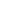Type Default Read Only Description [C#] `XRect` [Visual Basic] `XRect` Letter Size [0, 0, 612, 792]. No The current document page size.Notes

 This property reflects the size of the current page. It also determines the size of new pages created by the AddPage method. Note that changing the MediaBox does not change the current Rect. So typically you'll want to write code like this: [C#] ```theDoc.MediaBox.String = "0 0 200 300"; theDoc.Rect.String = theDoc.MediaBox.String;``` [Visual Basic] ```theDoc.MediaBox.String = "0 0 200 300" theDoc.Rect.String = theDoc.MediaBox.String``` Changing this property will change the size of pages created by subsequent calls to AddPage. However it will not change the size of the pages that have already been created. To change the size of the pages that have already been created you need to use the SetInfo method. For example: [C#] ```theDoc.SetInfo(theDoc.Page, "/MediaBox:Rect", "0 0 200 300");``` [Visual Basic] ```theDoc.SetInfo(theDoc.Page, "/MediaBox:Rect", "0 0 200 300")``` Similar methods can be used to control other page size measures such as the CropBox, BleedBox, TrimBox and ArtBox. For example: [C#] ```theDoc.SetInfo(theDoc.Page, "/CropBox:Rect", "20 20 180 280");``` [Visual Basic] ```theDoc.SetInfo(theDoc.Page, "/CropBox:Rect", "20 20 180 280")``` The default page size is often the one you'll want to use. However it may be that your PDFs are required to conform to a different page size. If this is the case you can simply specify the name of the size rather than the exact dimension. See the Rect.String property for details.Example

 The following code creates a PDF document containing three different pages each with a different size. [C#] ```Doc theDoc = new Doc(); theDoc.Page = theDoc.AddPage(); theDoc.MediaBox.String = "A4"; theDoc.Page = theDoc.AddPage(); theDoc.MediaBox.String = "B5"; theDoc.Page = theDoc.AddPage(); theDoc.Save(Server.MapPath("docmediabox.pdf")); theDoc.Clear();``` [Visual Basic] ```Dim theDoc As Doc = New Doc() theDoc.Page = theDoc.AddPage() theDoc.MediaBox.String = "A4" theDoc.Page = theDoc.AddPage() theDoc.MediaBox.String = "B5" theDoc.Page = theDoc.AddPage() theDoc.Save(Server.MapPath("docmediabox.pdf")) theDoc.Clear()```